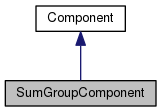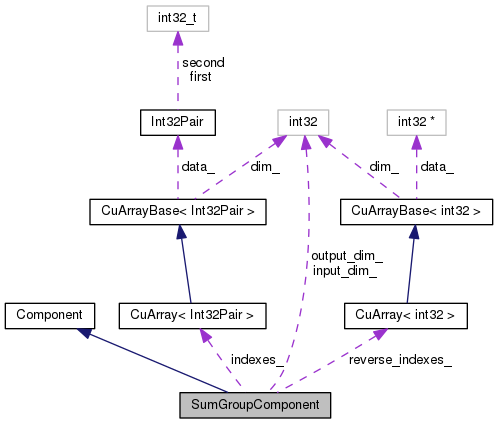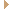SumGroupComponent Class Reference

SumGroupComponent is used to sum up groups of posteriors. More...

`#include <nnet-simple-component.h>`

Inheritance diagram for SumGroupComponent:[legend]
Collaboration diagram for SumGroupComponent:[legend]

## Public Member Functions

virtual int32 InputDim () const
Returns input-dimension of this component. More...

virtual int32 OutputDim () const
Returns output-dimension of this component. More...

void Init (const std::vector< int32 > &sizes)

void Init (int32 input_dim, int32 output_dim)

void GetSizes (std::vector< int32 > *sizes) const

virtual void InitFromConfig (ConfigLine *cfl)
Initialize, from a ConfigLine object. More...

SumGroupComponent ()

virtual std::string Type () const
Returns a string such as "SigmoidComponent", describing the type of the object. More...

virtual int32 Properties () const
Return bitmask of the component's properties. More...

virtual void * Propagate (const ComponentPrecomputedIndexes *indexes, const CuMatrixBase< BaseFloat > &in, CuMatrixBase< BaseFloat > *out) const
Propagate function. More...

virtual void Backprop (const std::string &debug_info, const ComponentPrecomputedIndexes *indexes, const CuMatrixBase< BaseFloat > &in_value, const CuMatrixBase< BaseFloat > &, const CuMatrixBase< BaseFloat > &out_deriv, void *memo, Component *to_update, CuMatrixBase< BaseFloat > *in_deriv) const
Backprop function; depending on which of the arguments 'to_update' and 'in_deriv' are non-NULL, this can compute input-data derivatives and/or perform model update. More...

virtual ComponentCopy () const
Copies component (deep copy). More...

virtual void Read (std::istream &is, bool binary)
Read function (used after we know the type of the Component); accepts input that is missing the token that describes the component type, in case it has already been consumed. More...

virtual void Write (std::ostream &os, bool binary) const
Write component to stream. More...Public Member Functions inherited from Component
virtual void StoreStats (const CuMatrixBase< BaseFloat > &in_value, const CuMatrixBase< BaseFloat > &out_value, void *memo)
This function may store stats on average activation values, and for some component types, the average value of the derivative of the nonlinearity. More...

virtual void ZeroStats ()
Components that provide an implementation of StoreStats should also provide an implementation of ZeroStats(), to set those stats to zero. More...

virtual void GetInputIndexes (const MiscComputationInfo &misc_info, const Index &output_index, std::vector< Index > *desired_indexes) const
This function only does something interesting for non-simple Components. More...

virtual bool IsComputable (const MiscComputationInfo &misc_info, const Index &output_index, const IndexSet &input_index_set, std::vector< Index > *used_inputs) const
This function only does something interesting for non-simple Components, and it exists to make it possible to manage optionally-required inputs. More...

virtual void ReorderIndexes (std::vector< Index > *input_indexes, std::vector< Index > *output_indexes) const
This function only does something interesting for non-simple Components. More...

virtual ComponentPrecomputedIndexesPrecomputeIndexes (const MiscComputationInfo &misc_info, const std::vector< Index > &input_indexes, const std::vector< Index > &output_indexes, bool need_backprop) const
This function must return NULL for simple Components. More...

virtual std::string Info () const

virtual void Scale (BaseFloat scale)
This virtual function when called on – an UpdatableComponent scales the parameters by "scale" when called by an UpdatableComponent. More...

virtual void Add (BaseFloat alpha, const Component &other)
This virtual function when called by – an UpdatableComponent adds the parameters of another updatable component, times some constant, to the current parameters. More...

virtual void DeleteMemo (void *memo) const
This virtual function only needs to be overwritten by Components that return a non-NULL memo from their Propagate() function. More...

virtual void ConsolidateMemory ()
This virtual function relates to memory management, and avoiding fragmentation. More...

Component ()

virtual ~Component ()

## Private Member Functions

KALDI_DISALLOW_COPY_AND_ASSIGN (SumGroupComponent)

## Private Attributes

CuArray< Int32Pairindexes_

CuArray< int32reverse_indexes_

int32 input_dim_

int32 output_dim_Static Public Member Functions inherited from Component
static ComponentReadNew (std::istream &is, bool binary)
Read component from stream (works out its type). Dies on error. More...

static ComponentNewComponentOfType (const std::string &type)
Returns a new Component of the given type e.g. More...

## Detailed Description

SumGroupComponent is used to sum up groups of posteriors.

It's used to introduce a kind of Gaussian-mixture-model-like idea into neural nets. This is basically a degenerate case of MixtureProbComponent; we had to implement it separately to be efficient for CUDA (we can use this one regardless whether we have CUDA or not; it's the normal case we want anyway).

There are two forms of initialization in a config file: one where the number of elements are specified for each group individually as a vector, and one where only the total input dimension and the output dimension (number of groups) is specified. The second is used when all groups have the same size.

Definition at line 1053 of file nnet-simple-component.h.

## ◆ SumGroupComponent()

 SumGroupComponent ( )
inline

Definition at line 1064 of file nnet-simple-component.h.

1064 { }

## ◆ Backprop()

 void Backprop ( const std::string & debug_info, const ComponentPrecomputedIndexes * indexes, const CuMatrixBase< BaseFloat > & in_value, const CuMatrixBase< BaseFloat > & out_value, const CuMatrixBase< BaseFloat > & out_deriv, void * memo, Component * to_update, CuMatrixBase< BaseFloat > * in_deriv ) const
virtual

Backprop function; depending on which of the arguments 'to_update' and 'in_deriv' are non-NULL, this can compute input-data derivatives and/or perform model update.

Parameters
 [in] debug_info The component name, to be printed out in any warning messages. [in] indexes A pointer to some information output by this class's PrecomputeIndexes function (will be NULL for simple components, i.e. those that don't do things like splicing). [in] in_value The matrix that was given as input to the Propagate function. Will be ignored (and may be empty) if Properties()&kBackpropNeedsInput == 0. [in] out_value The matrix that was output from the Propagate function. Will be ignored (and may be empty) if Properties()&kBackpropNeedsOutput == 0 [in] out_deriv The derivative at the output of this component. [in] memo This will normally be NULL, but for component types that set the flag kUsesMemo, this will be the return value of the Propagate() function that corresponds to this Backprop() function. Ownership of any pointers is not transferred to the Backprop function; DeleteMemo() will be called to delete it. [out] to_update If model update is desired, the Component to be updated, else NULL. Does not have to be identical to this. If supplied, you can assume that to_update->Properties() & kUpdatableComponent is nonzero. [out] in_deriv The derivative at the input of this component, if needed (else NULL). If Properties()&kBackpropInPlace, may be the same matrix as out_deriv. If Properties()&kBackpropAdds, this is added to by the Backprop routine, else it is set. The component code chooses which mode to work in, based on convenience.

Implements Component.

Definition at line 3551 of file nnet-simple-component.cc.

References CuMatrixBase< Real >::CopyCols(), and NVTX_RANGE.

3558  {
3559  NVTX_RANGE("SumGroupComponent::Backprop");
3560  in_deriv->CopyCols(out_deriv, reverse_indexes_);
3561 }
#define NVTX_RANGE(name)
Definition: cu-common.h:143

## ◆ Copy()

 Component * Copy ( ) const
virtual

Copies component (deep copy).

Implements Component.

Definition at line 3499 of file nnet-simple-component.cc.

3499  {
3500  SumGroupComponent *ans = new SumGroupComponent();
3501  ans->indexes_ = indexes_;
3502  ans->reverse_indexes_ = reverse_indexes_;
3503  ans->input_dim_ = input_dim_;
3504  ans->output_dim_ = output_dim_;
3505  return ans;
3506 }

## ◆ GetSizes()

 void GetSizes ( std::vector< int32 > * sizes ) const

Definition at line 3522 of file nnet-simple-component.cc.

References rnnlm::i, and KALDI_ASSERT.

3522  {
3523  std::vector<Int32Pair> indexes;
3524  indexes_.CopyToVec(&indexes);
3525  sizes->resize(indexes.size());
3526  for (size_t i = 0; i < indexes.size(); i++) {
3527  (*sizes)[i] = indexes[i].second - indexes[i].first;
3528  if (i == 0) { KALDI_ASSERT(indexes[i].first == 0); }
3529  else { KALDI_ASSERT(indexes[i].first == indexes[i-1].second); }
3530  KALDI_ASSERT(indexes[i].second > indexes[i].first);
3531  (*sizes)[i] = indexes[i].second - indexes[i].first;
3532  }
3533 }
void CopyToVec(std::vector< T > *dst) const
This function resizes *dst if needed.
Definition: cu-array-inl.h:177
#define KALDI_ASSERT(cond)
Definition: kaldi-error.h:185

## ◆ Init() [1/2]

 void Init ( const std::vector< int32 > & sizes )

Definition at line 3440 of file nnet-simple-component.cc.

References rnnlm::i, rnnlm::j, and KALDI_ASSERT.

3440  {
3441  KALDI_ASSERT(!sizes.empty());
3442  std::vector<Int32Pair> cpu_vec(sizes.size());
3443  std::vector<int32> reverse_cpu_vec;
3444  int32 cur_index = 0;
3445  for (size_t i = 0; i < sizes.size(); i++) {
3446  KALDI_ASSERT(sizes[i] > 0);
3447  cpu_vec[i].first = cur_index;
3448  cpu_vec[i].second = cur_index + sizes[i];
3449  cur_index += sizes[i];
3450  for (int32 j = cpu_vec[i].first; j < cpu_vec[i].second; j++)
3451  reverse_cpu_vec.push_back(i);
3452  }
3453  this->indexes_ = cpu_vec;
3454  this->reverse_indexes_ = reverse_cpu_vec;
3455  this->input_dim_ = cur_index;
3456  this->output_dim_ = sizes.size();
3457 }
kaldi::int32 int32
#define KALDI_ASSERT(cond)
Definition: kaldi-error.h:185

## ◆ Init() [2/2]

 void Init ( int32 input_dim, int32 output_dim )

Definition at line 3459 of file nnet-simple-component.cc.

References rnnlm::i, rnnlm::j, and KALDI_ASSERT.

3459  {
3460  const int32 num_groups = output_dim;
3461  KALDI_ASSERT(input_dim % num_groups == 0);
3462  const int32 group_size = input_dim / num_groups;
3463
3464  std::vector<Int32Pair> cpu_vec(num_groups);
3465  std::vector<int32> reverse_cpu_vec;
3466  int32 cur_index = 0;
3467  for (size_t i = 0; i < num_groups; i++) {
3468  cpu_vec[i].first = cur_index;
3469  cpu_vec[i].second = cur_index + group_size;
3470  cur_index += group_size;
3471  for (int32 j = cpu_vec[i].first; j < cpu_vec[i].second; j++)
3472  reverse_cpu_vec.push_back(i);
3473  }
3474  this->indexes_ = cpu_vec;
3475  this->reverse_indexes_ = reverse_cpu_vec;
3476  this->input_dim_ = input_dim;
3477  this->output_dim_ = num_groups;
3478 }
kaldi::int32 int32
#define KALDI_ASSERT(cond)
Definition: kaldi-error.h:185

## ◆ InitFromConfig()

 void InitFromConfig ( ConfigLine * cfl )
virtual

Initialize, from a ConfigLine object.

Parameters
 [in] cfl A ConfigLine containing any parameters that are needed for initialization. For example: "dim=100 param-stddev=0.1"

Implements Component.

Definition at line 3480 of file nnet-simple-component.cc.

3480  {
3481  std::vector<int32> sizes;
3482  bool has_sizes = cfl->GetValue("sizes", &sizes);
3483  if (has_sizes) {
3484  if (cfl->HasUnusedValues() || sizes.empty())
3485  KALDI_ERR << "Invalid initializer for layer of type "
3486  << Type() << ": \"" << cfl->WholeLine() << "\"";
3487  this->Init(sizes);
3488  } else { // each group has the same size
3489  int32 input_dim = -1, output_dim = -1;
3490  if (!cfl->GetValue("input-dim", &input_dim) ||
3491  !cfl->GetValue("output-dim", &output_dim) || cfl->HasUnusedValues()) {
3492  KALDI_ERR << "Invalid initializer for layer of type "
3493  << Type() << ": \"" << cfl->WholeLine() << "\"";
3494  }
3495  Init(input_dim, output_dim);
3496  }
3497 }
void Init(const std::vector< int32 > &sizes)
kaldi::int32 int32
virtual std::string Type() const
Returns a string such as "SigmoidComponent", describing the type of the object.
#define KALDI_ERR
Definition: kaldi-error.h:147

## ◆ InputDim()

 virtual int32 InputDim ( ) const
inlinevirtual

Returns input-dimension of this component.

Implements Component.

Definition at line 1055 of file nnet-simple-component.h.

References PnormComponent::input_dim_.

## ◆ KALDI_DISALLOW_COPY_AND_ASSIGN()

 KALDI_DISALLOW_COPY_AND_ASSIGN ( SumGroupComponent )
private

## ◆ OutputDim()

 virtual int32 OutputDim ( ) const
inlinevirtual

Returns output-dimension of this component.

Implements Component.

Definition at line 1056 of file nnet-simple-component.h.

## ◆ Propagate()

 void * Propagate ( const ComponentPrecomputedIndexes * indexes, const CuMatrixBase< BaseFloat > & in, CuMatrixBase< BaseFloat > * out ) const
virtual

Propagate function.

Parameters
 [in] indexes A pointer to some information output by this class's PrecomputeIndexes function (will be NULL for simple components, i.e. those that don't do things like splicing). [in] in The input to this component. Num-columns == InputDim(). [out] out The output of this component. Num-columns == OutputDim(). Note: output of this component will be added to the initial value of "out" if Properties()&kPropagateAdds != 0; otherwise the output will be set and the initial value ignored. Each Component chooses whether it is more convenient implementation-wise to add or set, and the calling code has to deal with it.
Returns
Normally returns NULL, but may return a non-NULL value for components which have the flag kUsesMemo set. This value will be passed into the corresponding Backprop routine.

Implements Component.

Definition at line 3544 of file nnet-simple-component.cc.

References CuMatrixBase< Real >::SumColumnRanges().

3546  {
3547  out->SumColumnRanges(in, indexes_);
3548  return NULL;
3549 }

## ◆ Properties()

 virtual int32 Properties ( ) const
inlinevirtual

Return bitmask of the component's properties.

These properties depend only on the component's type. See enum ComponentProperties.

Implements Component.

Definition at line 1066 of file nnet-simple-component.h.

1066 { return kSimpleComponent; }

 void Read ( std::istream & is, bool binary )
virtual

Read function (used after we know the type of the Component); accepts input that is missing the token that describes the component type, in case it has already been consumed.

Implements Component.

Definition at line 3508 of file nnet-simple-component.cc.

3508  {
3509  ExpectOneOrTwoTokens(is, binary, "<SumGroupComponent>", "<Sizes>");
3510  std::vector<int32> sizes;
3512
3513  std::string token;
3515  if (!(token == "<SumGroupComponent>" ||
3516  token == "</SumGroupComponent>")) {
3517  KALDI_ERR << "Expected </SumGroupComponent>, got " << token;
3518  }
3519  this->Init(sizes);
3520 }
void Init(const std::vector< int32 > &sizes)
void ReadToken(std::istream &is, bool binary, std::string *str)
ReadToken gets the next token and puts it in str (exception on failure).
Definition: io-funcs.cc:154
void ExpectOneOrTwoTokens(std::istream &is, bool binary, const std::string &token1, const std::string &token2)
This function is like ExpectToken but for two tokens, and it will either accept token1 and then token...
Definition: text-utils.cc:536
void ReadIntegerVector(std::istream &is, bool binary, std::vector< T > *v)
Function for reading STL vector of integer types.
Definition: io-funcs-inl.h:232
#define KALDI_ERR
Definition: kaldi-error.h:147

## ◆ Type()

 virtual std::string Type ( ) const
inlinevirtual

Returns a string such as "SigmoidComponent", describing the type of the object.

Implements Component.

Definition at line 1065 of file nnet-simple-component.h.

1065 { return "SumGroupComponent"; }

## ◆ Write()

 void Write ( std::ostream & os, bool binary ) const
virtual

Write component to stream.

Implements Component.

Definition at line 3535 of file nnet-simple-component.cc.

References kaldi::WriteIntegerVector(), and kaldi::WriteToken().

3535  {
3536  WriteToken(os, binary, "<SumGroupComponent>");
3537  WriteToken(os, binary, "<Sizes>");
3538  std::vector<int32> sizes;
3539  this->GetSizes(&sizes);
3540  WriteIntegerVector(os, binary, sizes);
3541  WriteToken(os, binary, "</SumGroupComponent>");
3542 }
void GetSizes(std::vector< int32 > *sizes) const
void WriteToken(std::ostream &os, bool binary, const char *token)
The WriteToken functions are for writing nonempty sequences of non-space characters.
Definition: io-funcs.cc:134
void WriteIntegerVector(std::ostream &os, bool binary, const std::vector< T > &v)
Function for writing STL vectors of integer types.
Definition: io-funcs-inl.h:198

## ◆ indexes_

 CuArray indexes_
private

Definition at line 1086 of file nnet-simple-component.h.

Referenced by SumGroupComponent::Copy().

## ◆ input_dim_

 int32 input_dim_
private

Definition at line 1089 of file nnet-simple-component.h.

Referenced by SumGroupComponent::Copy().

## ◆ output_dim_

 int32 output_dim_
private

Definition at line 1090 of file nnet-simple-component.h.

Referenced by SumGroupComponent::Copy().

## ◆ reverse_indexes_

 CuArray reverse_indexes_
private

Definition at line 1088 of file nnet-simple-component.h.

Referenced by SumGroupComponent::Copy().

The documentation for this class was generated from the following files: